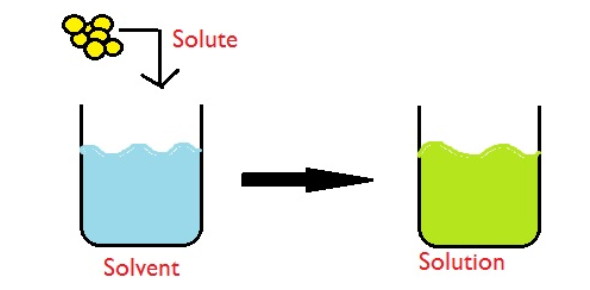# Solutions And Mixtures

28 Questions | Attempts: 3888
ShareSettingsThis is a quiz over how well you know 9th grade physical science containing solutions and mixtures.

• 1.
The oceans are an example of a(n)______solution.
• A.

Gaseous

• B.

Liquid

• C.

Solid

• D.

Anhydrous

• 2.
In a solution, the substance which is being dissolved is the_____.
• A.

Solvent

• B.

Solute

• C.

Liquid

• D.

Gas

• 3.
A solution that contains all the solute it can hold at a given temperature is _____.
• A.

Supersaturated

• B.

Dilute

• C.

Saturated

• D.

Unsaturated

• 4.
The solute that is found in the greatest amount in ocean water is______.
• A.

H20

• B.

KCl

• C.

NaCl

• D.

CuSO4

• 5.
A substance that does not conduct an electric current when it forms a solution is_____.
• A.

A polar substance

• B.

A nonpolar substance

• C.

A nonelectrolyte

• D.

An electrolyte

• 6.
The process by which the positive and negative ions of an ionic compound sperate in water is called____.
• A.

Dissociation

• B.

Ionization

• C.

Saturation

• D.

Polarity

• 7.
When a gas is dissolved in a liquid, the gas dissolves faster if the liquid is ____.
• A.

Heated

• B.

Cooled

• C.

An electrolyte

• D.

Under low pressure

• 8.
Increasing the surface area of a solid _____.
• A.

Causes the solid to ionize

• B.

Slows the rate of solution

• C.

Speeds the rate of solution

• D.

Has no effect on the rate of solution

• 9.
Any method of removing salts from ocean water is called____.
• A.

Desalination

• B.

Distillation

• C.

Evaporation

• D.

Condensation

• 10.
The process in which fresh water is obtained from ocean water that has been evaporated and condensed is called ____.
• A.

Ionization

• B.

Dissociation

• C.

Distillation

• D.

The freeze method

• 11.
The concentration of a solution that contains much solute in the solvent could be described as _____.
• A.

Polar

• B.

Concentrated

• C.

Unsaturated

• D.

Dilute

• 12.
An alloy is an example of a _____.
• A.

Gaseous solution

• B.

Liquid solution

• C.

Dilute solution

• D.

Solid solution

• 13.
Adding more solute to a solvent _____.
• A.

Decreases its boiling point

• B.

Increases its freezing point

• C.

Increases its boiling point

• D.

Does not affect its boiling point

• 14.
When placed in water, some covalent compounds _____.
• A.

Ionize

• B.

• C.

Form protons

• D.

Dissociate

• 15.
Which of the following will speed up the dissolving of a solid solute in water?
• A.

Cool the solution

• B.

Stir the solution

• C.

Grind up the solvent

• D.

Freeze the solute

• 16.
Which of the following combinations would increase the solubility of a gas in a liquid?
• A.

Decrease the pressure and decrease the temperature

• B.

Decrease the pressure and increase the temperature

• C.

Increase the pressure and decrease the temperature

• D.

Increase the pressure and increase the temperature

• 17.
Solutions of electrolytes and metals are ____.
• A.

Insulators

• B.

Conductors

• C.

Polar

• D.

Negative

• 18.
The amount of solute that can be dissolved in a specific amount of solvent at a given temperature is its _____.
• A.

Concentration

• B.

Density

• C.

Dilution

• D.

Solubility

• 19.
A molecule that is positively charged on one end and negatively charged on the other end is ____.
• A.

Polar

• B.

Magnetic

• C.

Nonpolar

• D.

• 20.
__________ means that a substance is physically combined and can be separated into different parts.
• 21.
__________ means that a substance is chemically combined and can not be separated.
• 22.
The ______ is the substance being dissolved.
• 23.
The _____ is what you're dissolving something in.
• 24.
________ means there is a very small amount of solute.
• 25.
_____ means that the solvent can dissolve more and has NOT reached its limit.

## Related TopicsBack to top
×

Wait!
Here's an interesting quiz for you.